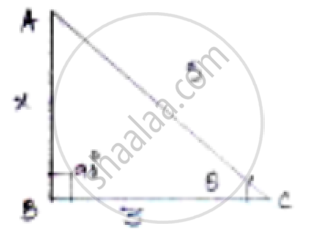Share

# If Cos Theta = 3/5, Find the Value of (Sin Theta - 1/(Tan Theta))/(2 Tan Theta) - CBSE Class 10 - Mathematics

#### Question

if cos theta = 3/5, find the value of (sin theta - 1/(tan theta))/(2 tan theta)

#### Solution

We know that cos theta = "𝑎𝑑𝑗𝑎𝑐𝑒𝑛𝑡 𝑠𝑖𝑑𝑒"/"ℎ𝑦𝑝𝑜𝑡𝑒𝑛𝑢𝑠𝑒"Let us consider right angled Δle ABC

Let x be the opposite side, By applying Pythagoras theorem

𝐴𝐶2 = 𝐴𝐵2 + 𝐵𝐶2

25 = 𝑥2 + 9

𝑥2 = 16 ⇒ 𝑥 = 4

sin theta = (AB)/(AC) = 4/5

tan theta = (AB)/(BC) = 4/3

Substitute sin 𝜃, tan 𝜃 in equation we get

(sin theta 1/(tan theta))/(2 tan theta) = (4/5 3/4)/(2 xx 4/3)

= ((16 - 15)/20)/(8/3) = (1/20)/(8/3)

=  1/20 xx  3/4 = 3/160

Is there an error in this question or solution?

#### APPEARS IN

Solution If Cos Theta = 3/5, Find the Value of (Sin Theta - 1/(Tan Theta))/(2 Tan Theta) Concept: Trigonometric Ratios.
S